## What is 1/2, 3/4, and 5/48 ordered least to greatest

Question

What is 1/2, 3/4, and 5/48 ordered least to greatest

in progress 0
5 months 2021-08-13T02:12:14+00:00 2 Answers 4 views 0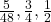Step-by-step explanation:

Given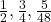Required

Order from least to greatest

To ease the process, we first convert the fractions to decimal: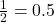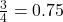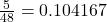By comparison:

0.104167 is less than 0.5 and 0.5 is less than 0.75

So, we have: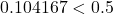and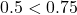So, from the least to greatest:

0.104167, 0.5, 0.75

Convert  to fraction5/48, 1/2 and 3/4

Step-by-step explanation:

You need to make a common denominator for all the numbers first.

1/2 is equal to 24/48

-multiply both sides by 24 because 48 divided by 2 is 24

3/4 equals 36/48

-multiply both sides by 12 because 48 divided by 4 equals 12

so you get:

24/48 (1/2)

36/48 (3/4)

and 5/48

now you order them:

5/48

24/48

36/48

and then you change them back to their original form:

5/48

1/2

3/4